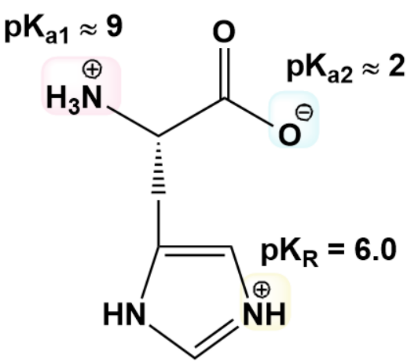Clutch Prep is now a part of Pearson
Ch. 3 - Amino AcidsWorksheetSee all chapters

# Amino Acids and Henderson-Hasselbalch

See all sections
Sections
Amino Acid Groups
Amino Acid Three Letter Code
Amino Acid One Letter Code
Amino Acid Configuration
Essential Amino Acids
Nonpolar Amino Acids
Aromatic Amino Acids
Polar Amino Acids
Charged Amino Acids
How to Memorize Amino Acids
Zwitterion
Non-Ionizable Vs. Ionizable R-Groups
Isoelectric Point
Isoelectric Point of Amino Acids with Ionizable R-Groups
Titrations of Amino Acids with Non-Ionizable R-Groups
Titrations of Amino Acids with Ionizable R-Groups
Amino Acids and Henderson-Hasselbalch

Concept #1: Applying Henderson-Hasselbalch to Amino Acids

Example #1: Example of Applying Henderson-Hasselbalch to Amino Acids

Practice: At pH 11.8, what is the % of protonated amino group in the R-group of Lysine. (Lysine’s pKR = 10.8)?

Practice: Draw Glu & calculate the % of -COOH in the R-group of Glu at pH 3.2. (Glu pK  R = 4.1).

Practice: Draw Asp & calculate the pH at which two thirds (2/3) of Asp’s R-group is dissociated. (Asp pKR = 3.9).

Practice: Draw Arg & calculate the pH at which 23% of Arg’s R-group is dissociated. (Arg pK  R = 12.5).

Practice: What is the ratio of [conjugate base] to [conjugate acid] for each of Histidine’s three ionizable groups at pH 7?A) Amino group ratio: ________________

B) Carboxyl group ratio: ________________

C) R-group ratio: ________________

D) Use the ratios above to determine the average net charge of the ionizable groups & the entire His molecule.

1. Net charge of Amino groups: ____________

2. Net charge of Carboxyl groups: ____________

3. Net charge of R-groups: ____________

4. Net charge of His: ____________ (Hint: sum previous 3 charges).